# Excel Alternatives to VLOOKUP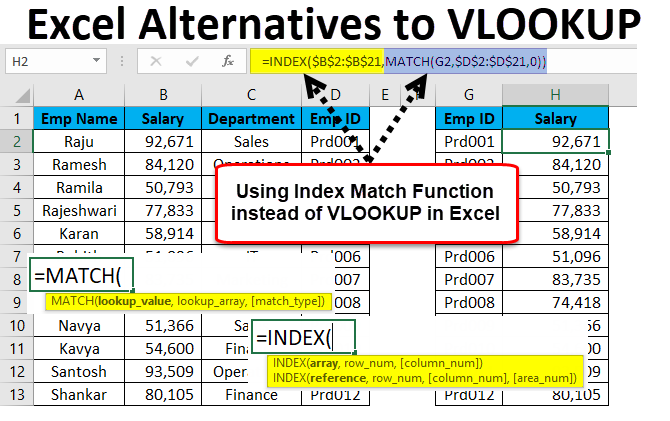• Alternatives to VLOOKUP
• Examples of INDEX and MATCH Function with VLOOKUP Limitation
• How to Use VLOOKUP Alternatives in Excel?

## Alternatives to VLOOKUP

Excel is full of formulas, rows, and columns. Vlookup is one of the important formulas in excel. You do not know Alternative to VLOOKUP formula in excel as an excellent user but you know the formula well.

However, if you think knowing the VLOOKUP formula is the ultimate thing in excel then you must change your thought immediately (I felt knowing VLOOKUP is the ultimate thing in excel early on in my career).

Once you start progressing to the advanced level you will start feeling the limitations of the VLOOKUP formula. I don’t know whether you are aware or not VLOOKUP can fetch you the data from left to right only. There are instances where I wanted to fetch the data from right to left but VLOOKUP failed to help me in those cases. I was forced to rearrange my data to get the job done in favor of me.

However, it is not an end because we have few alternatives to come over the limitations of the VLOOKUP in excel.

### Examples of INDEX and MATCH Function with VLOOKUP Limitation

These two are the alternatives to VLOOKUP in excel. For Alternative to VLOOKUP we need to remember the column number in case of many columns but in these formulas, we need not remember anything, we just need to understand the logic of the formula.

INDEX Formula: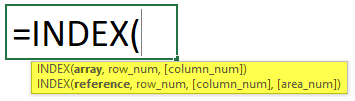• Array: What is the array value you want to fetch?
• Row Num: From which row number you are trying to fetch the data.
• Column Num: From which column number you are trying to fetch the data.

Let us look at some examples of Excel Alternatives to VLOOKUP.

### Index Function – Example #1

I have a simple zone-wise sales table.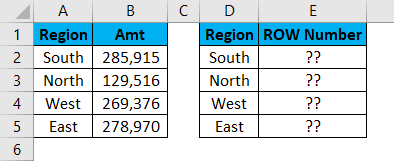I can fetch the data by using VLOOKUP but here I will use INDEX formula. Open the formula in E2 cell.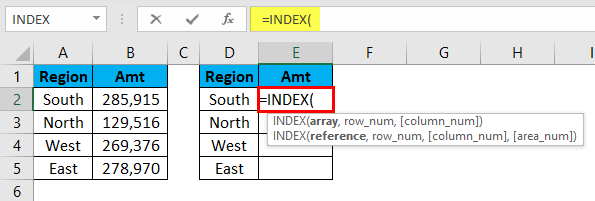Now select the required result column values. Here my required values are in from B2 to B5. I will select the range as B2:B5 and lock it.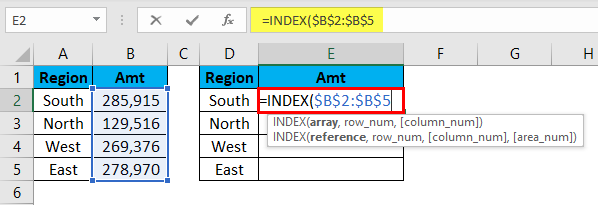Now I need to mention the Row Number. For the South region, the row number is 2.

Now I need to mention from which column we are trying to fetch the data. In this case is the first column because we have selected only one column in the array argument.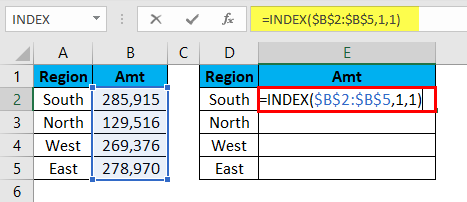INDEX will fetch me the data for the SOUTH region.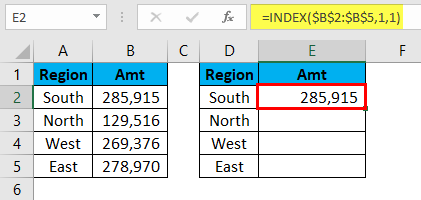Wow!!! INDEX has done the trick for me.

However, the problem will start now. When I copy and paste the formula to the below cells I need to change the row number as the cell changes. In the current cell row number is 1 and when I moved to the next cell it has to be 2. INDEX does not take the row incremental number automatically.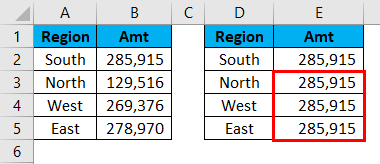We can automate this task by using the MATCH function.

### MATCH Function – Example #2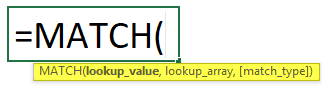It will return the row number of a selected value in the supplied range.

• Lookup Value: What is the lookup value you are trying to find the row number?
• Lookup Array: From which range you are trying to find the row number.
• Match Type: What kind of result you want to search. Whether it is the Approximate match below the lookup value (1) or Exact match (0) or approximate match above the lookup value (-1).

Now I will use the same data from the above example. I will try to look out the row number for each region.Open the formula in E2 cell and select the lookup value as D2 cell value.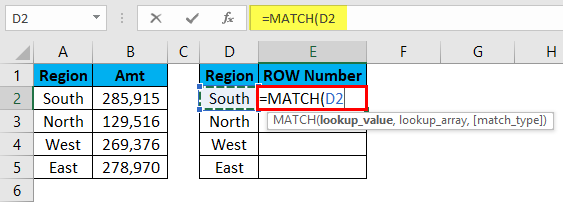Now lookup array is from which table you are trying to know the row number. Our array table is from A2 to A5.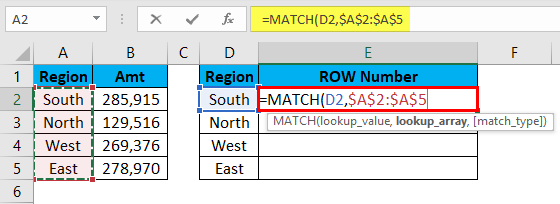Match type should be exact so I will mention 0 as the argument.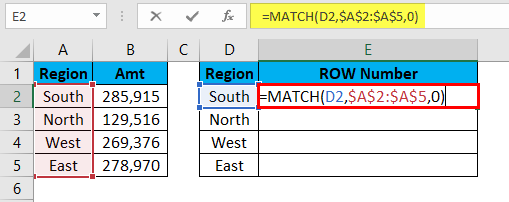I got the row numbers for each region.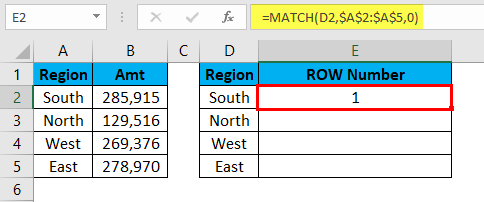Drag and drop the formula to remaining cells.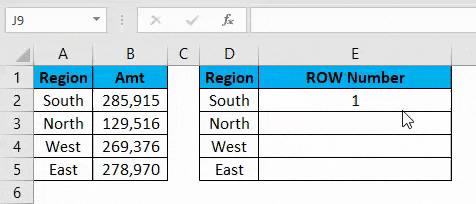### Limitation of VLOOKUP – Example #3

VLOOKUP always work from left to right. Now, look at the below data.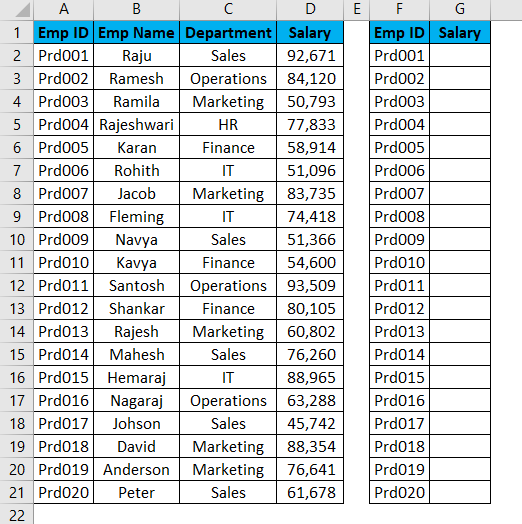I need to extract salary data from the range A1 to D21. I can do this by applying a VLOOKUP function.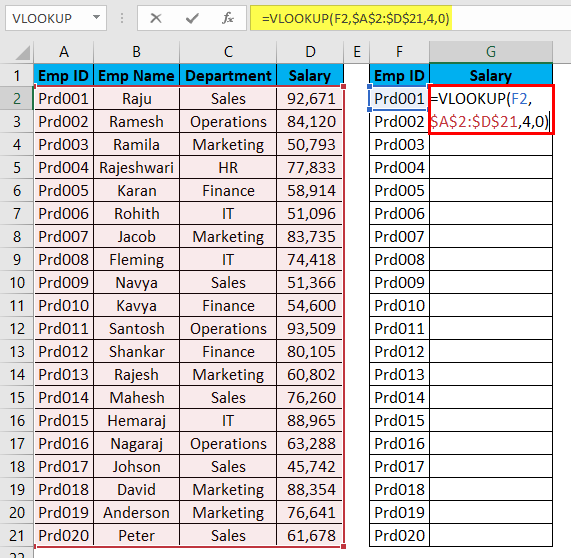The Result will be as below: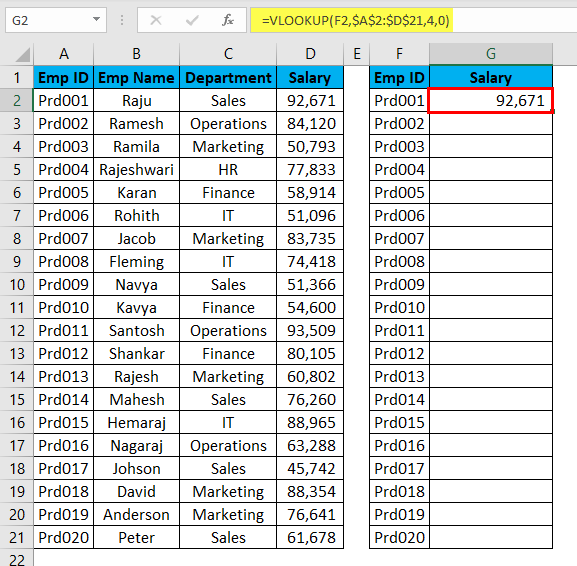Drag and drop the formula to remaining cells.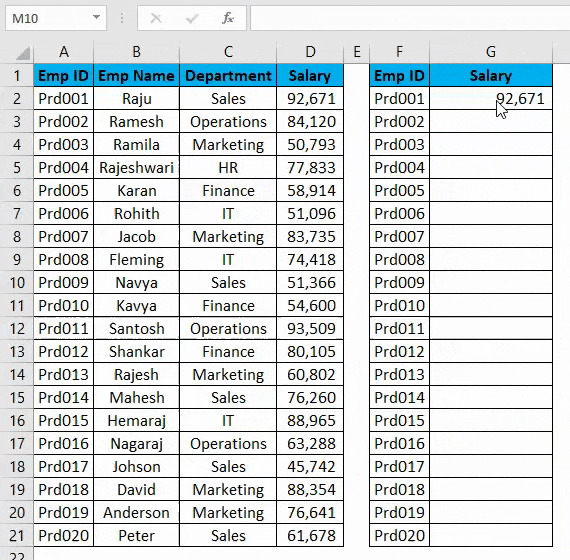At this point in time, VLOOKUP is doing the job for me. But now look at the below data structure.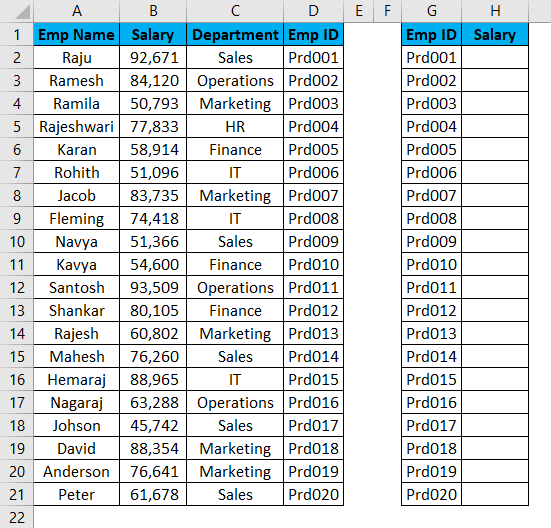Based on the EMP ID I need to extract the data but EMP ID is there at the very right end of the actual data. VLOOKUP starts to fade away in these cases.

So we have an excel alternative to VLOOKUP called INDEX & MATCH function.

### How to Use Alternative to VLOOKUP in Excel?

Alternative to VLOOKUP is very simple and easy. Let’s understand how to use alternatives to VLOOKUP in excel with some examples.

### INDEX + MATCH Function as Alternative to VLOOKUP – Example #1

Now take the same table from the above example.Open the INDEX formula in H2 cell.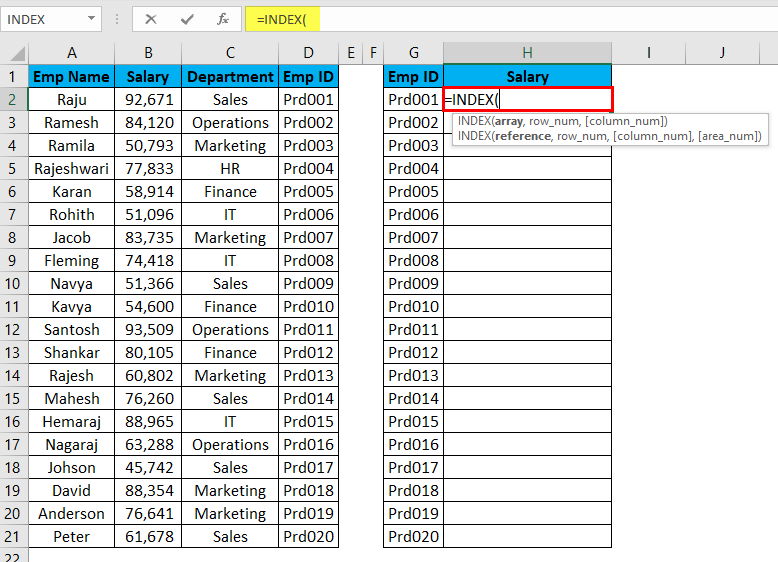An array is nothing but what is the result you are trying to find. Here we are trying to find the salary value, so select the entire salary range.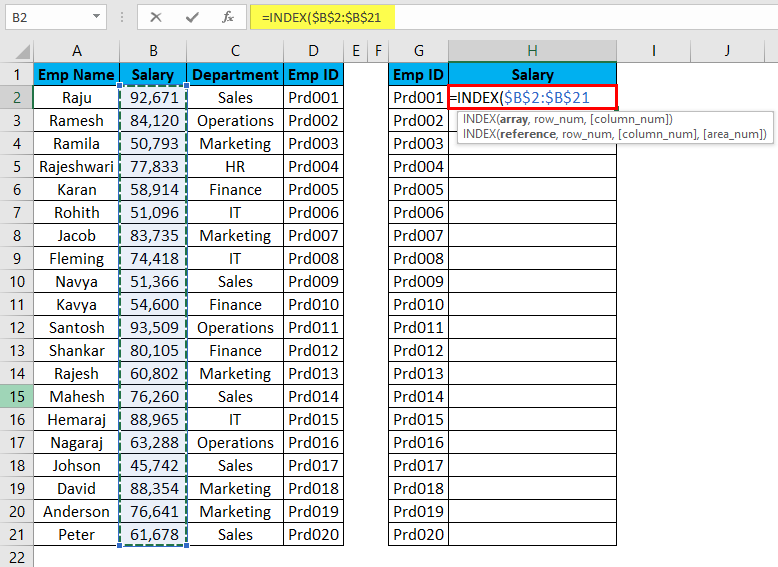Now we need to mention the row number. In the MATCH function example, we have learned MATCH function can give us the row number. So I will use a MATCH function inside the INDEX function.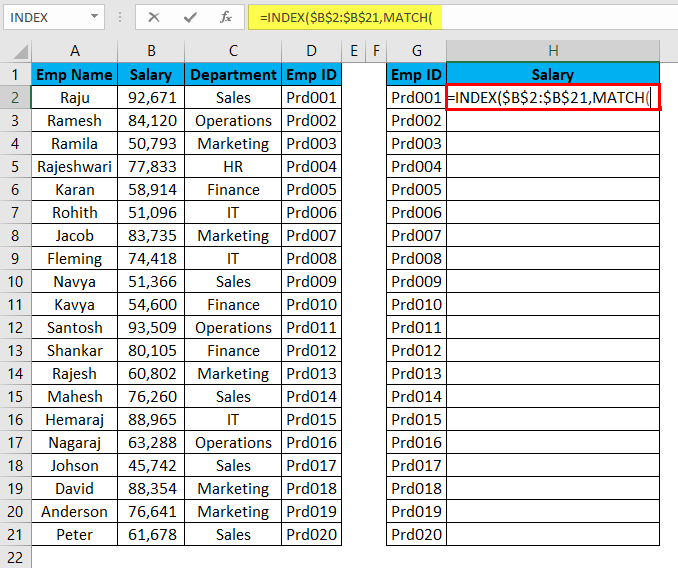LOOKUP means on what basis I am trying to find the row number. Here for EMP ID, I am trying to find the row number, so lookup value is EMP ID.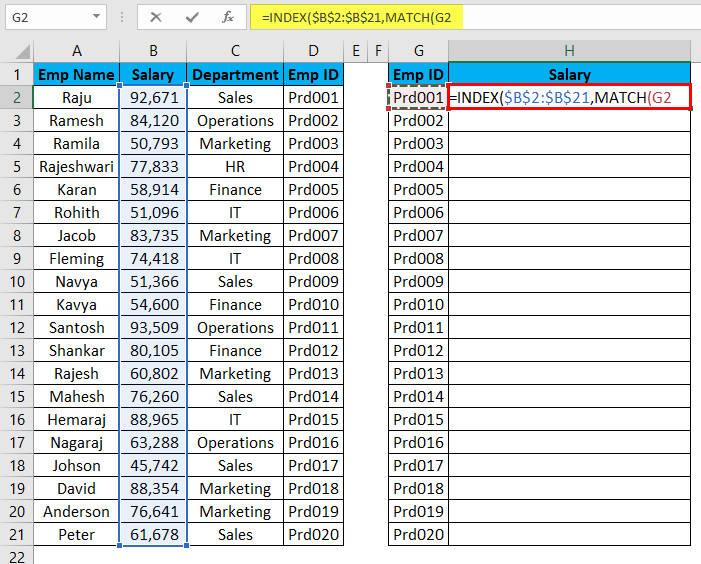Now look up array is nothing but it is the main table lookup value column.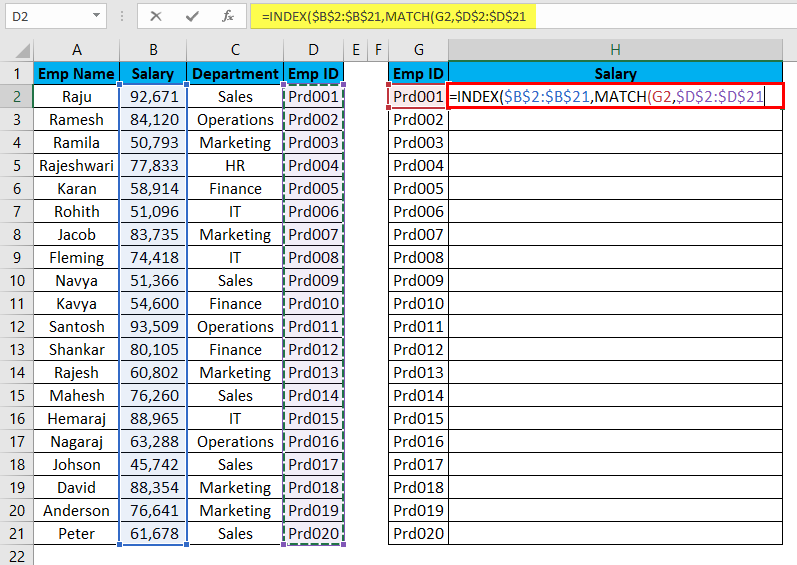Now finally match type should be the exact match so mention 0 as the value.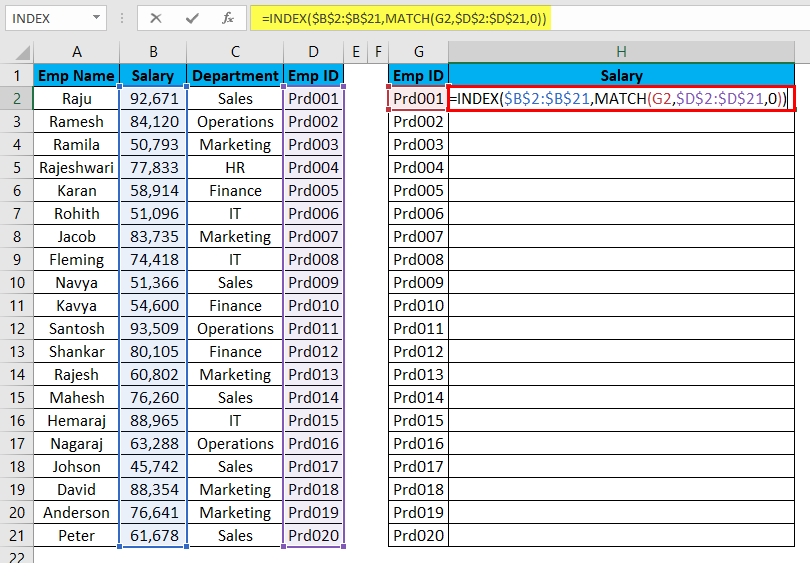The Result will be as below: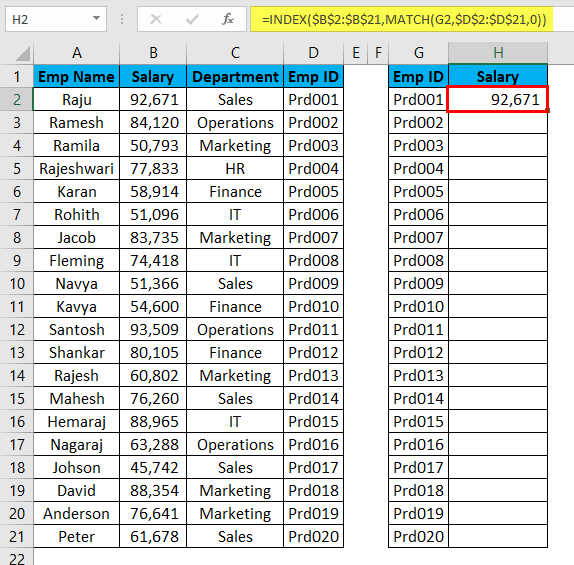Yessssss!!!!!!! INDEX + MATCH is working exactly like the VLOOKUP but can fetch the data from anywhere to anywhere.

Drag and drop the formula to remaining cells.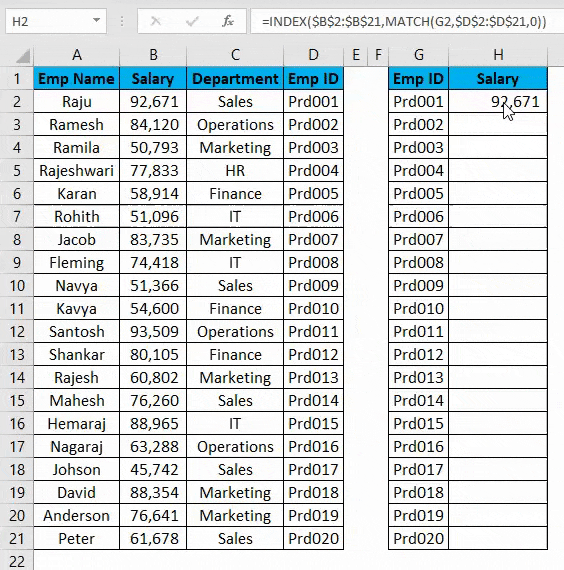### LOOKUP Function as Alternative to VLOOKUP – Example #2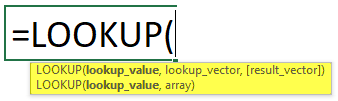LOOKUP itself is a built-in function in excel.

• Lookup Value: It is the value on the basis you are looking for the result.
• Lookup Vector: It is the Lookup value range in the main table.
• Result Vector: It is the result column in the main table.

Take the same data from the previous example.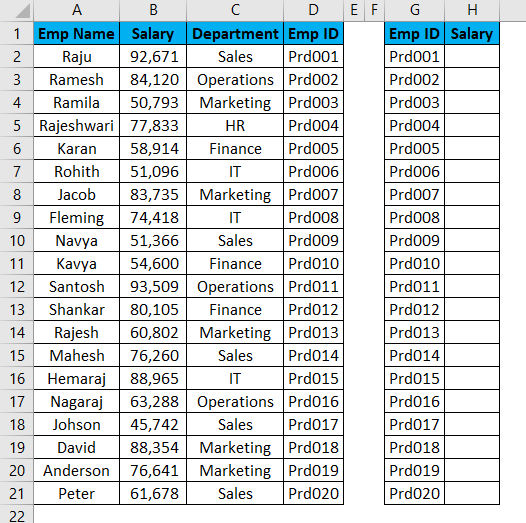Open the LOOKUP formula in H2 cell and select the lookup value as G2.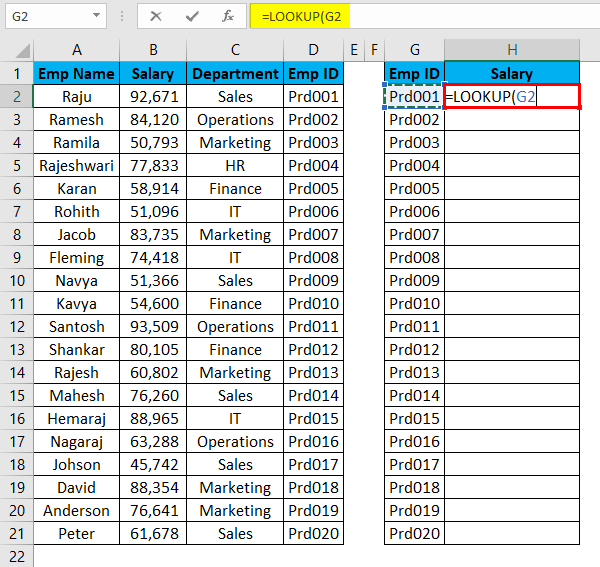Now we need to select the lookup vector as D2 to D21 in the main table.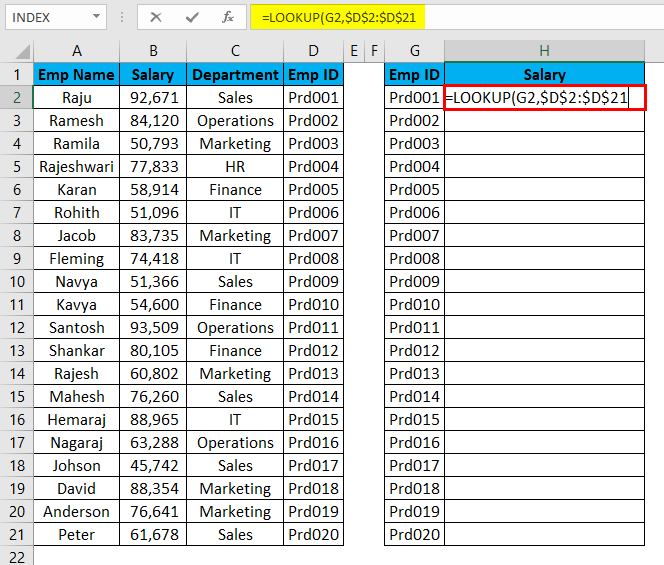Now finally the result vector should be the result column we are trying to extract which is B2 to B21.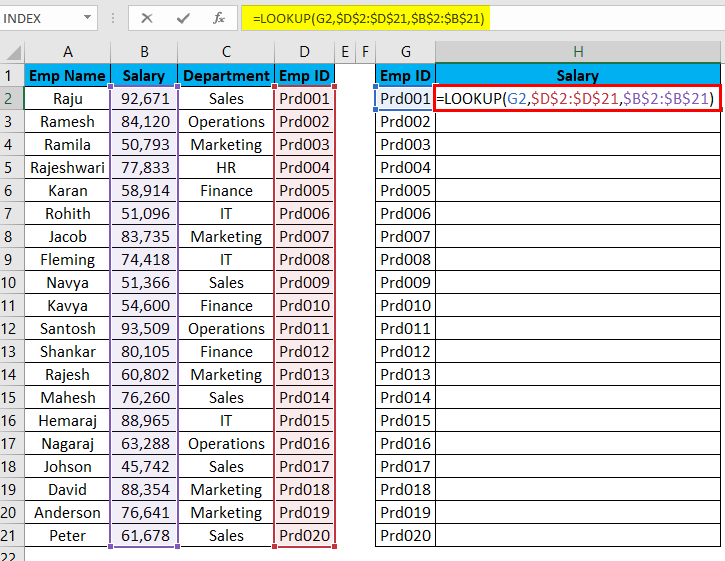After entering the formula hit the enter key, and we will get the result.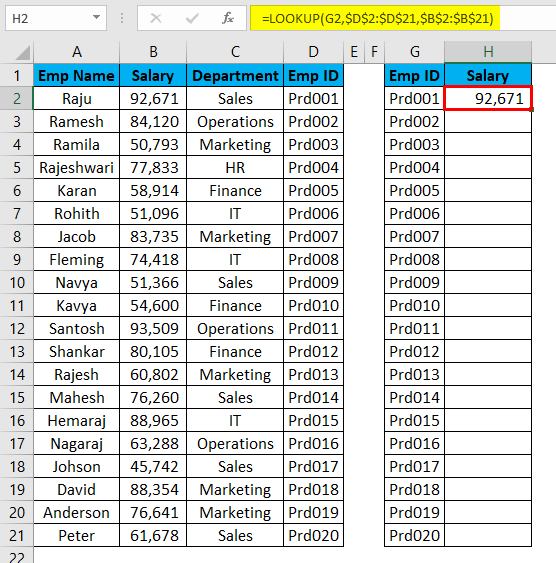Drag and drop the formula to remaining cells.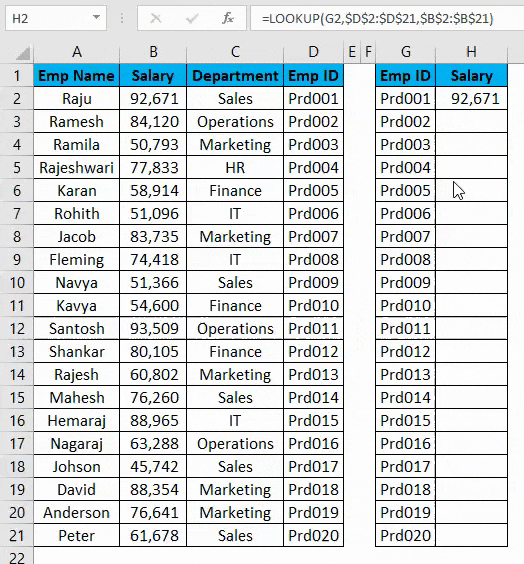### Things to Remember about Excel Alternatives to VLOOKUP

• VLOOKUP can only work from left to right.
• MATCH function will return the row number.
• INDEX + MATCH and LOOKUP functions do not require column number, unlike VLOOKUP, require column number to fetch the data even though the required column is already selected.
• Data structure does not matter for INDEX + MATCH and LOOKUP functions. But for VLOOKUP data structure matters.

You can download these Alternatives to VLOOKUP Excel Template here – Alternatives to VLOOKUP Excel Template

### Recommended Articles

This has been a guide to Alternatives to VLOOKUP in Excel. Here we discuss the examples of excel alternatives to VLOOKUP such as INDEX, MATCH, and LOOKUP along with practical examples and downloadable excel template. You can also go through our other suggested articles –

1. VLOOKUP Function in Excel
2. Tutorials for MATCH Function in Excel
3. How to Use LOOKUP in Excel?
4. IFERROR with VLOOKUP in Excel

The post Excel Alternatives to VLOOKUP appeared first on EDUCBA.

This post first appeared on Best Online Training & Video Courses | EduCBA, please read the originial post: here

# Share the post

Excel Alternatives to VLOOKUP

×